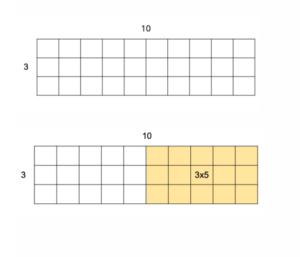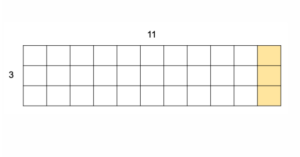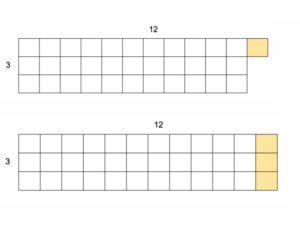# Resources

## Developing Number Sense with Number Strings

By Avery McNiff

April 14, 2021

What are Number Strings?

A number string is a series of carefully structured and related math problems that develop an understanding of number relationships and operations (Fosnot & Dolk, 2001). The practice engages students in problem solving and provides opportunities for students to participate in math discourse.

Number strings are a flexible, adaptable, and short (15-20 minutes) activity that can serve as a daily warm up with whole classes or small groups. Teachers can design number strings around a range of mathematical topics, from addition to algebra.

Number Strings in Practice

A small group of fifth grade students gather around a table. The teacher begins the lesson by asking students to brainstorm strategies they know for multiplication. One student shares, “counting by 10s, 5s, any number,” which the teacher labels as skip counting. They review repeated addition (e.g., 5 + 5 + 5 = 5×3), doubling (e.g., 3×10 = 2 x (3×5)  = 3×5+3×5), and arrays. Arrays are a model consisting of rows and columns that can be used to explore and understand properties of multiplication and division. A student adds, “breaking it down,” which is a newer strategy for the group and also the focus of the day’s number string. The teacher has designed this particular string around “breaking it down,” or the distributive property, and uses arrays, a familiar model to these students, to represent the strategy.

After the initial brainstorm, the teacher writes the first problem on the board.

3×5

“Give me a thumb’s up when you have it,” the teacher says before providing ample wait time for students to think.

After everyone has a “quiet thumb,” the teacher calls on students to share an answer, prompting them to explain their strategy or build off of the strategies of others. Students solved the problem by skip counting by 3, skip counting by 5, and repeated addition. The teacher represents their thinking on the board by drawing a closed 3×5 array. She uses the image to illustrate the idea of skip counting (3,6,9,12,15 or 5,10,15) and repeated addition (5+5+5 or 3+3+3+3+3).

Next, the teacher writes the following helper problem, which builds upon the first, highlights doubling, sets up a conversation around place value, and serves as an anchor for illustrating the distributive property:3×10

A student shares the answer 30. When asked “How?” the student answers, “by adding 10+10+10.” After documenting the strategy, the teacher brings the discussion back to the model by pointing to the 3×5 array and asking, “What will the array look like if I’m going to make it represent this problem? Are you imagining what is going to happen?”

Students share that it will get bigger. The teacher then draws the 3×10 array and prompts students to look for the 3×5 array within it, drawing a connection to the previous problem and highlighting doubling.3×11

The teacher cues students to first think about the array: “What would it look like now?” At this point in the lesson, students know that the array has to be bigger, but by how much? With the guidance of a student pointing to the array on the board, the teacher adds another row of 3 to the 3×10 array. A student shares her strategy of multiplying 3×1 and 3×1, which leads to a conversation around place value. “Is the 1 really a 1 or is it a 10?” the teacher asks before using the concrete example of money, ten dollar bills and one dollar bills, to further illustrate this concept.3×12References and Resources

Fosnot, C. T., & Dolk, M. (2001). Young mathematicians at work, 2: Constructing
multiplication and division. Heinemann.

Lambert, R., Imm, K., & Williams, D. A. (2017). Number strings: Daily computational fluency.Avery McNiff is a Ph.D. student in Special Education at the University of California Santa Barbara. Her personal research interests focus on math instruction for students with learning disabilities, particularly dyslexia. She has worked as a graduate student researcher on projects related to math participation and engagement, UDL and design thinking, and UDL training for preservice teachers. Prior to beginning her studies at UCSB, Avery taught elementary and middle school math and technology at Landmark School.

## Strategies to Check Out

Number Strings Lesson Plan

Ideas for Implementing Number Strings Online

## WoodinMath.com

Explore WoodinMath for additional strategies to help students visualize mathematics concepts.